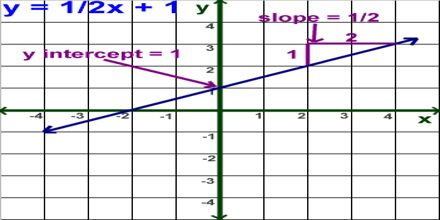Mathematic

# Slope and Y-interceptThe aim of this lecture is to present on Slope and Y-intercept. In the equation of a straight line (when the equation is written as “y = mx + b”), the slope is the number “m” that is multiplied on the x, and “b” is the y-intercept, where the line crosses the y-axis. This useful form of the line equation is sensibly named the “slope-intercept form”. The equation of any straight line, called a linear equation, can be written as: y = mx + b, where m is the slope of the line and b is the y-intercept. The y-intercept of this line is the value of y at the point where the line crosses the y axis.